# 三十代殘兵游勇

## 六月 4, 2016

### 20160605 2016春アニメ「Macross Delta、 リルリルフェアリル、迷家、プリパラ S3」

Filed under: 總合批評 — handelshieh @ 週六 2016/06/04 23:46:30 GMT+0800

4+4+3+5+5  3+4+3+4+3=38「Macross Delta 002」
5+4+3+5+4  3+4+3+5+4=40「Macross Delta 003」
4+4+3+3+4  3+4+3+3+3=34「Macross Delta 004」
4+5+3+5+4  3+4+3+3+3=37「Macross Delta 005」
4+4+3+4+4  3+3+4+3+3=35「Macross Delta 006」
4+3+3+4+4  3+3+3+3+3=33「Macross Delta 007」
3+4+3+5+3  4+4+3+3+3=35「Macross Delta 008」
4+4+4+4+3  3+3+3+3+3=34「Macross Delta 009」

4+4+3+3+3  3+5+3+3+3=32「迷家‐マヨヒガ‐ 009」
4+3+3+3+3(+-1)  3+3+3+3+4=32「迷家‐マヨヒガ‐ 010」

4+3+3+4+4  3+4+3+3+3=34「 リルリルフェアリル〜妖精のドア〜 017」

4+4+3+3+4  3+3+3+3+3=33「 プリパラ S3 009 (098)」

2016春アニメ「 うしおととら 3季、セーラームーンCrystal 3期、迷家、甲鉄城のカバネリ…」及其他

2016春季，個人標準「環境真實性比較」(依實際播出進度修改) ；過去連結一連結二
2016春季，個人標準「片頭尾評價列表」 ；過去連結一連結二連結三
2016春季新番+Plus 日本編 其二
2016春季新番+Plus 日本編 其一

4+4+3+5+5  3+4+3+4+3=38「Macross Delta 002」機遇回。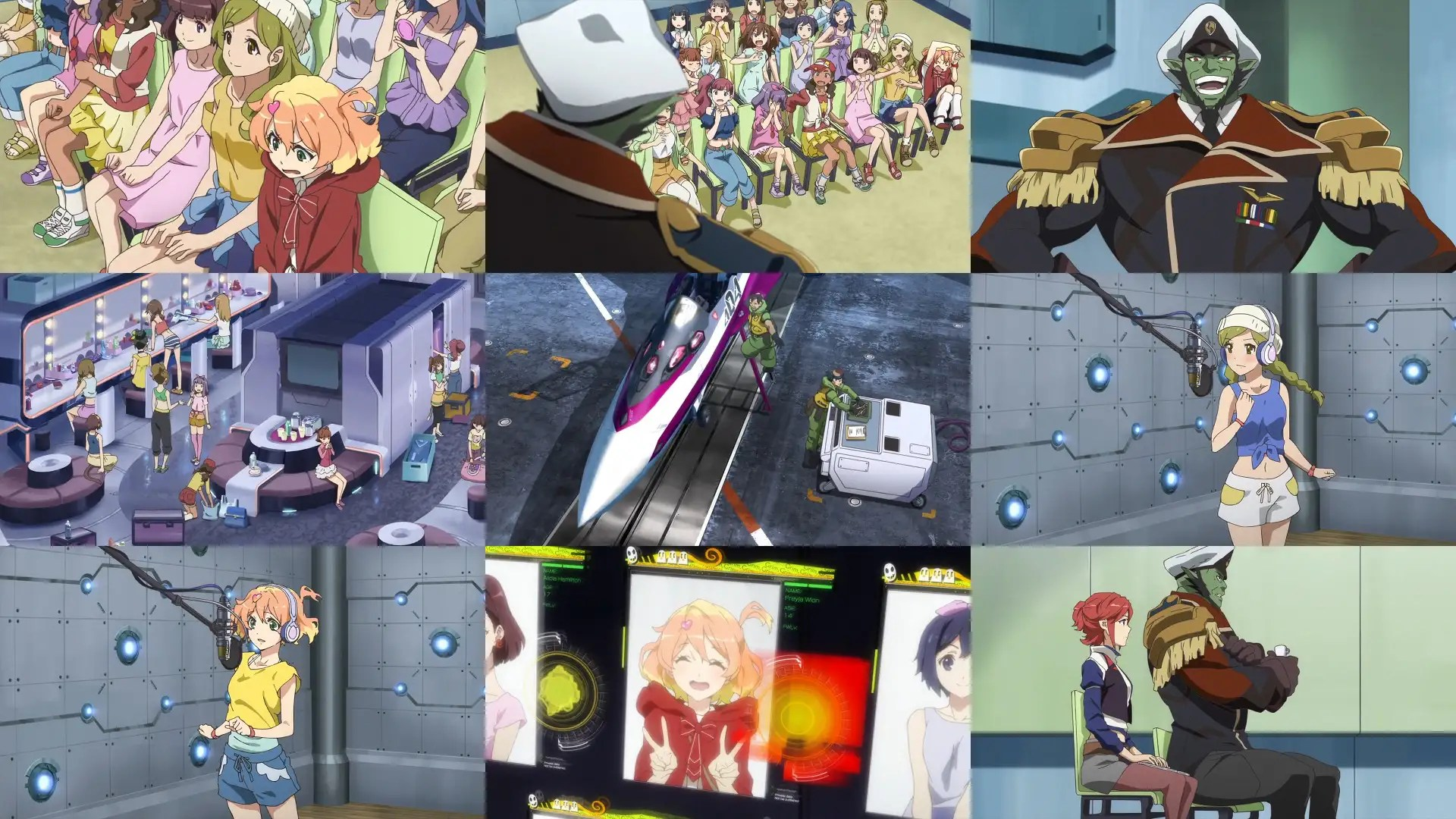5+4+3+5+4 3+4+3+5+4=40「Macross Delta 003」共鳴回。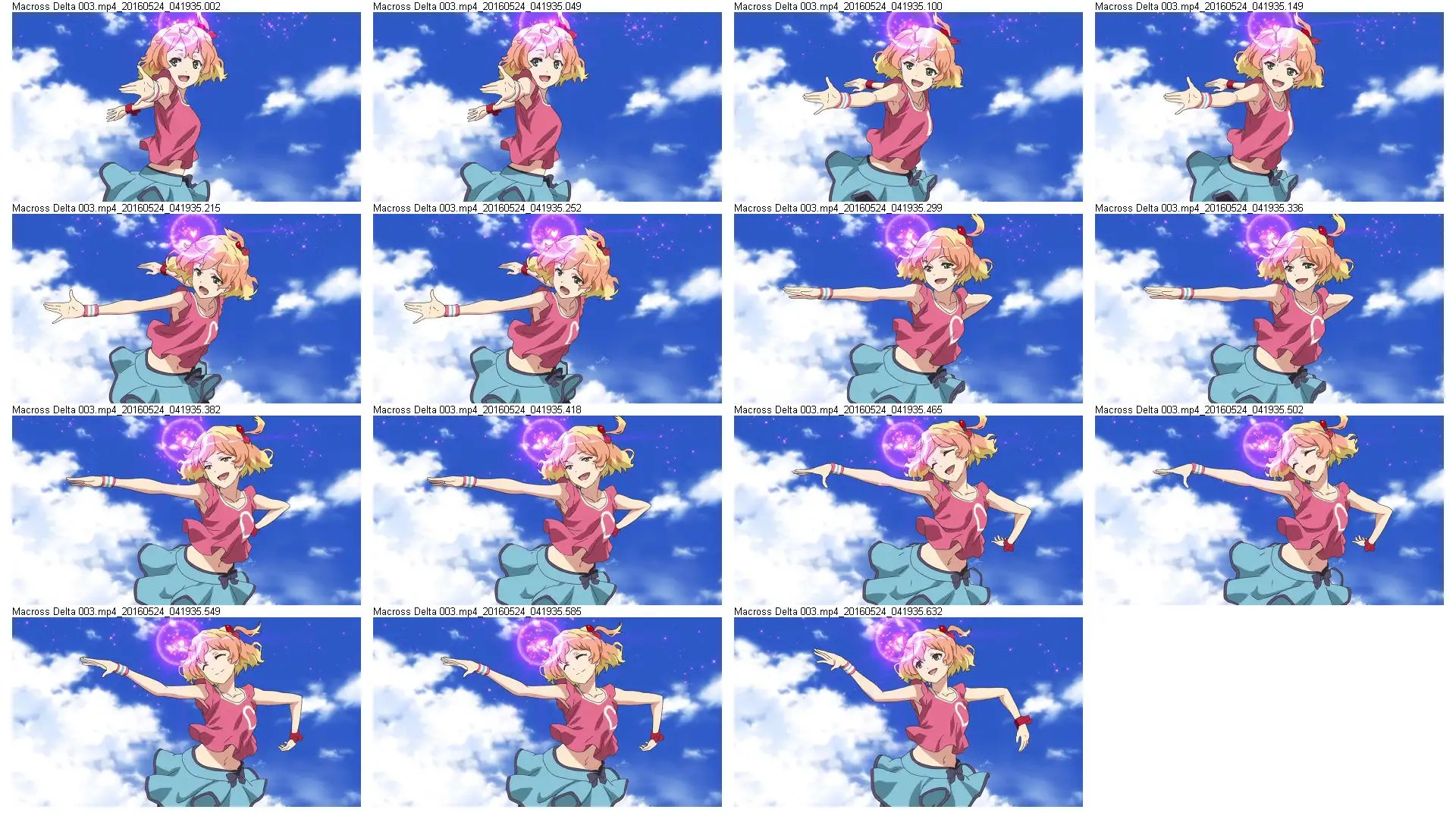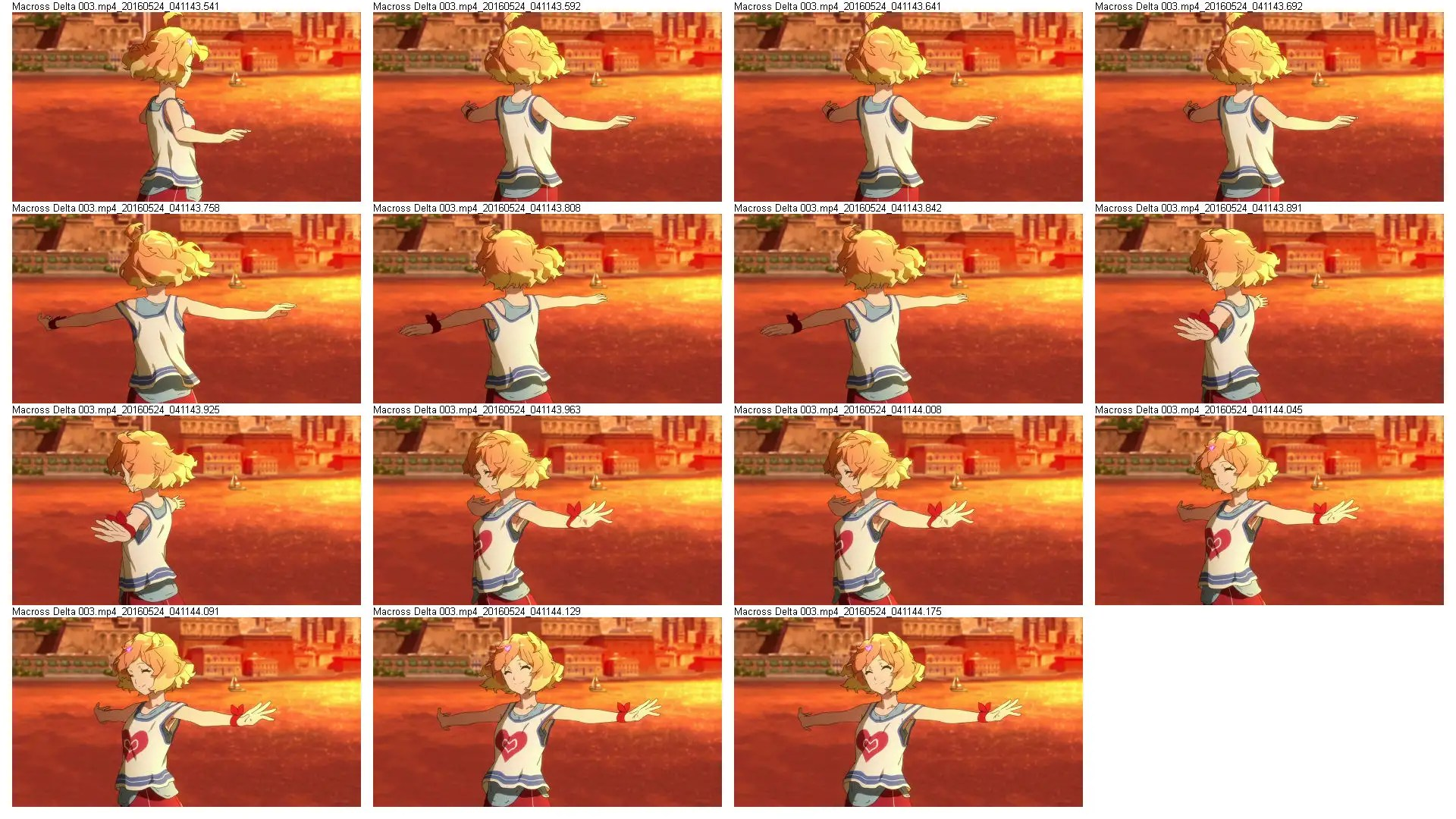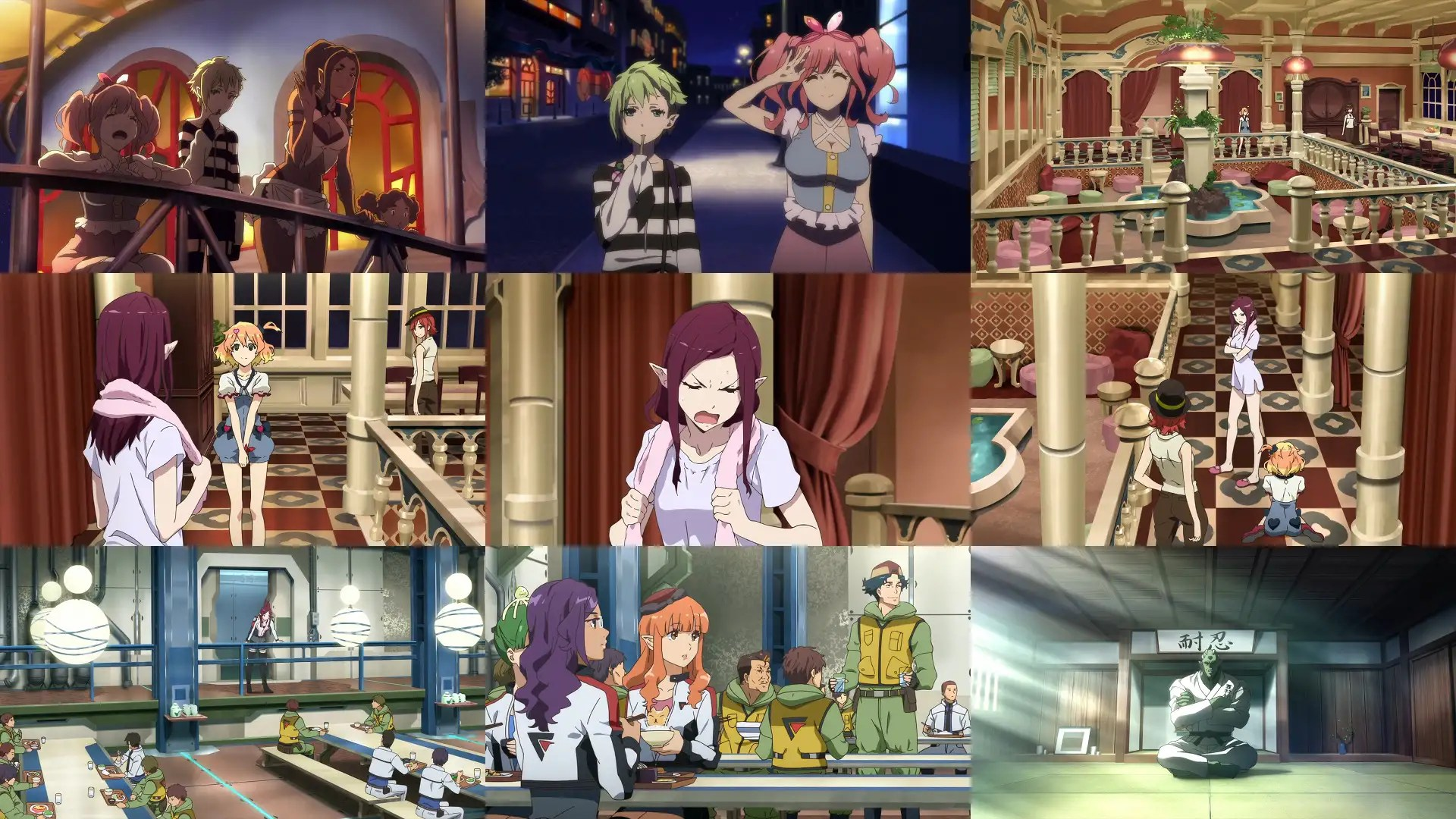4+4+3+3+4 3+4+3+3+3=34「Macross Delta 004」初Live與開戰宣言回。

マクロスF 即使播出至今近八年，ランカ・リー的初Live令人難忘，從天而降 (キラッ)是名場面，成功的單集重點演出。フレイア小妹顯然初Live機遇差了一些。反而是前幾集與男女主角共鬥交互影響共鳴的場景經典多了。

「歌はOOXX」其實這段很兒童向耶，我記得像アイカツ！還是プリリズ有過這樣的定義橋段。其實這新曲展露會，整個題材風格也偏向兒童偶像アニメ風，走可愛類型。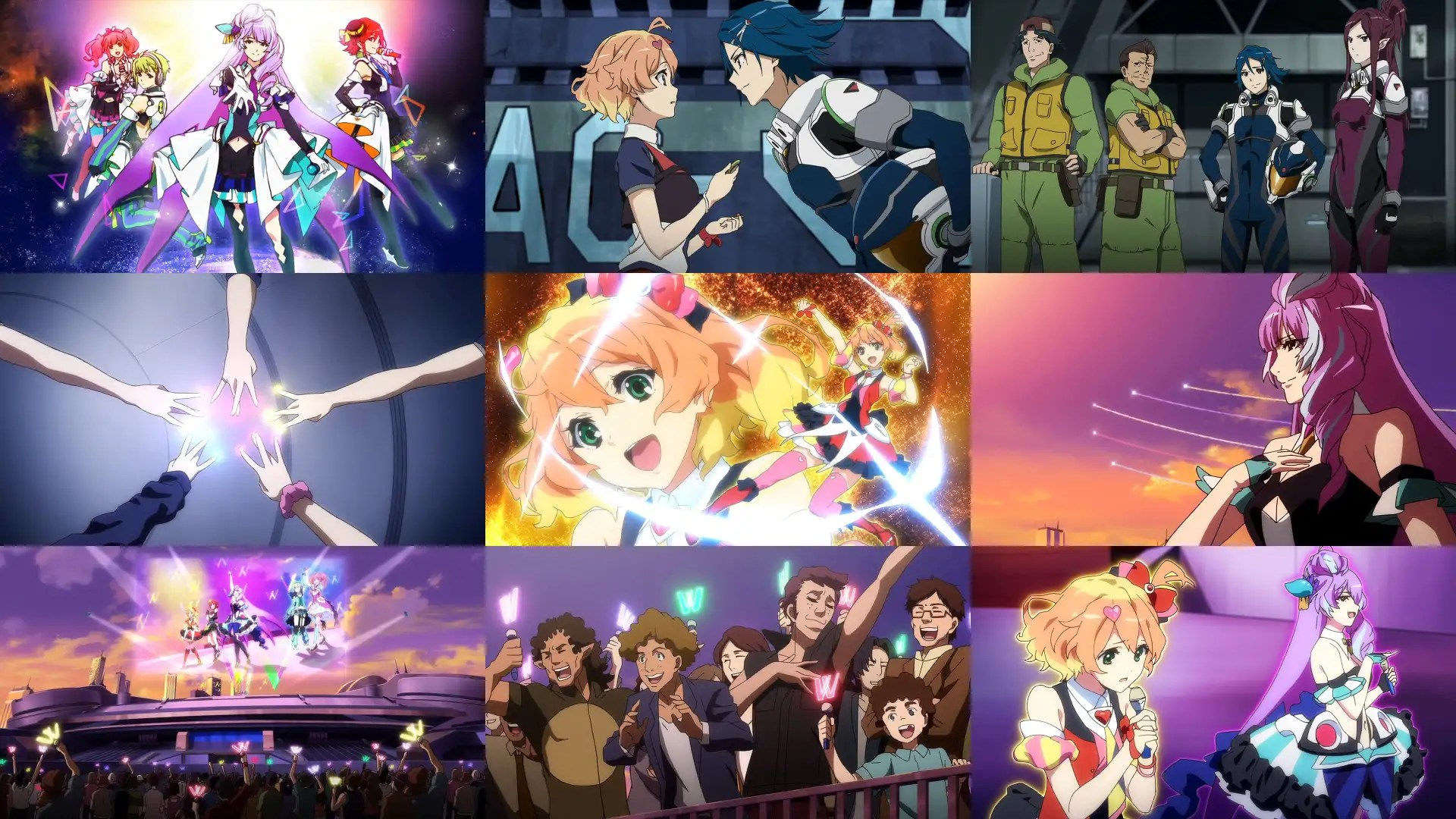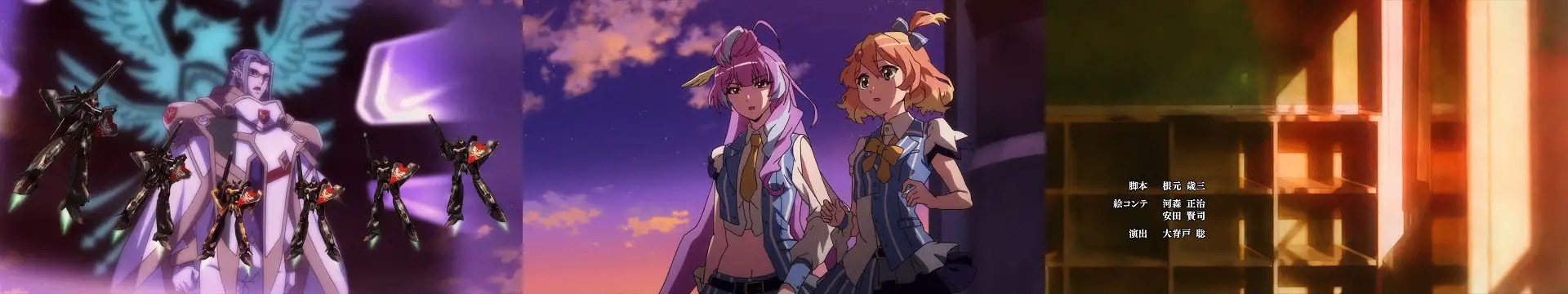4+5+3+5+4 3+4+3+3+3=37「Macross Delta 005」決心回。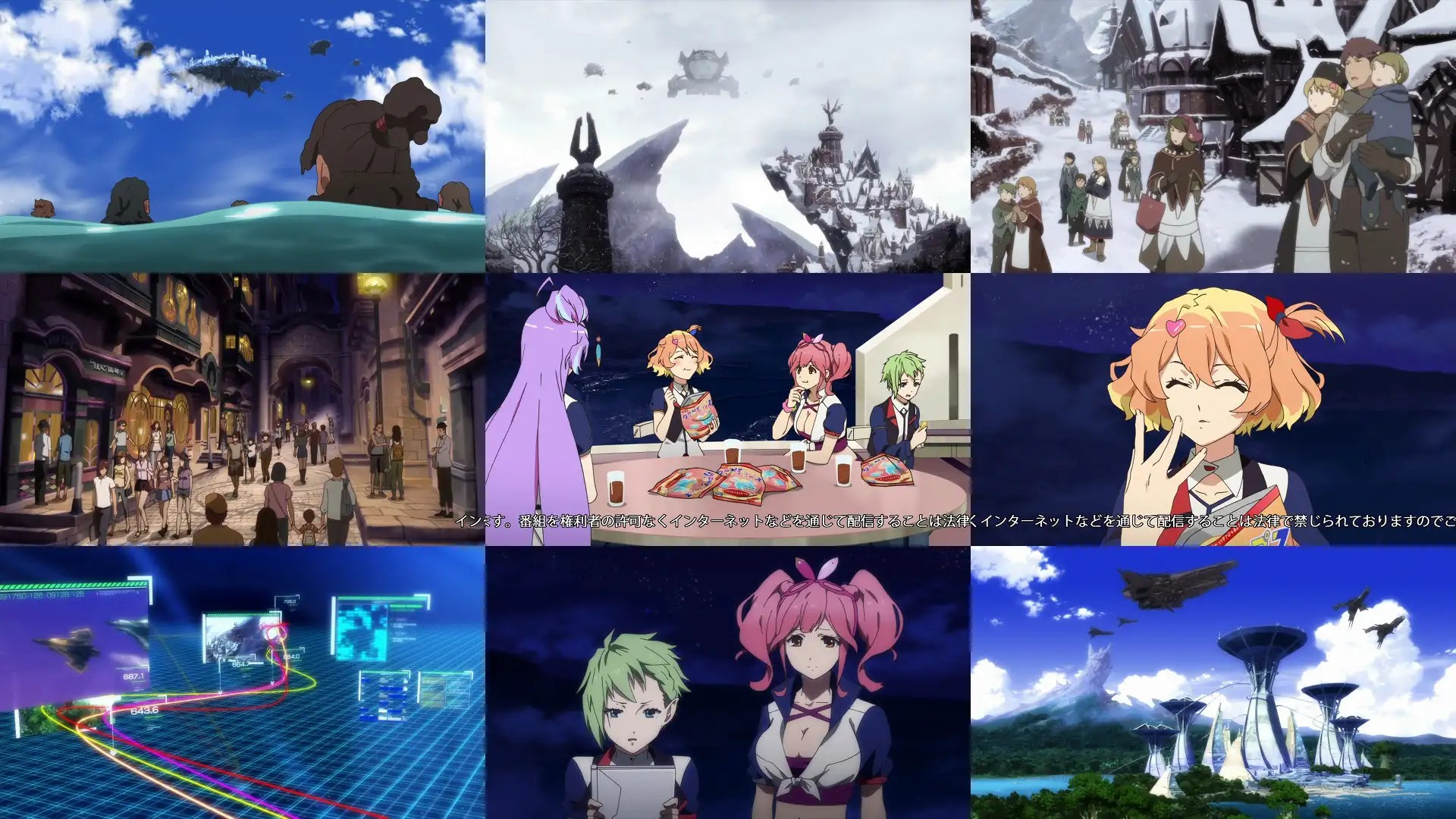4+4+3+4+4 3+3+4+3+3=35「Macross Delta 006」「為何而戰？」回。
3DCG模型戰機，出發前的腳部細節微控動作很棒。其實個人不太中意這段碎石帶？小行星帶？的戰鬥，畫面很雜亂，過於重視速度，而失去空間方向感。

3DCG在碎石帶？小行星帶？的戰鬥，個人認為還遠不如ダンボール戦機W的宇宙戰。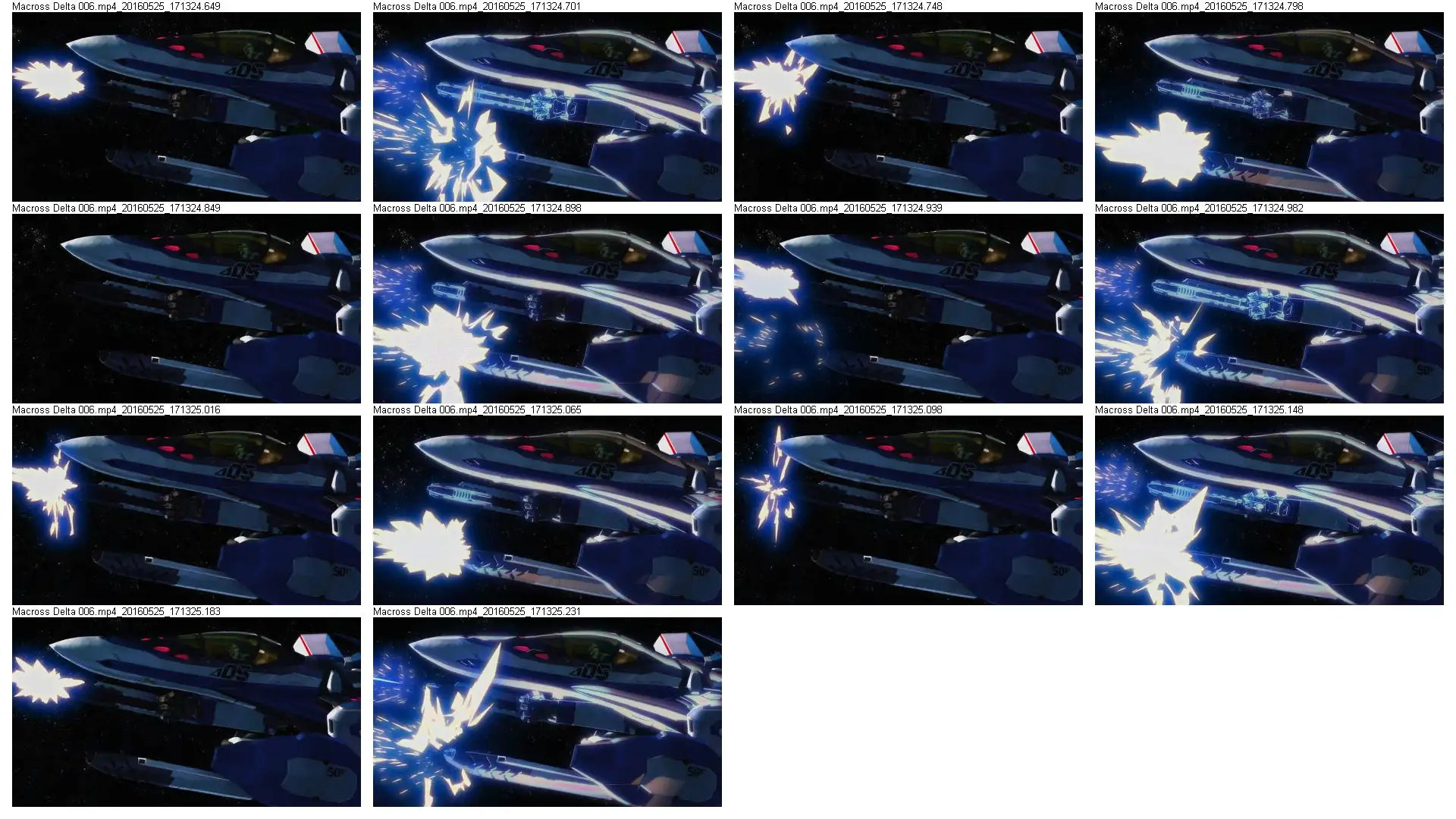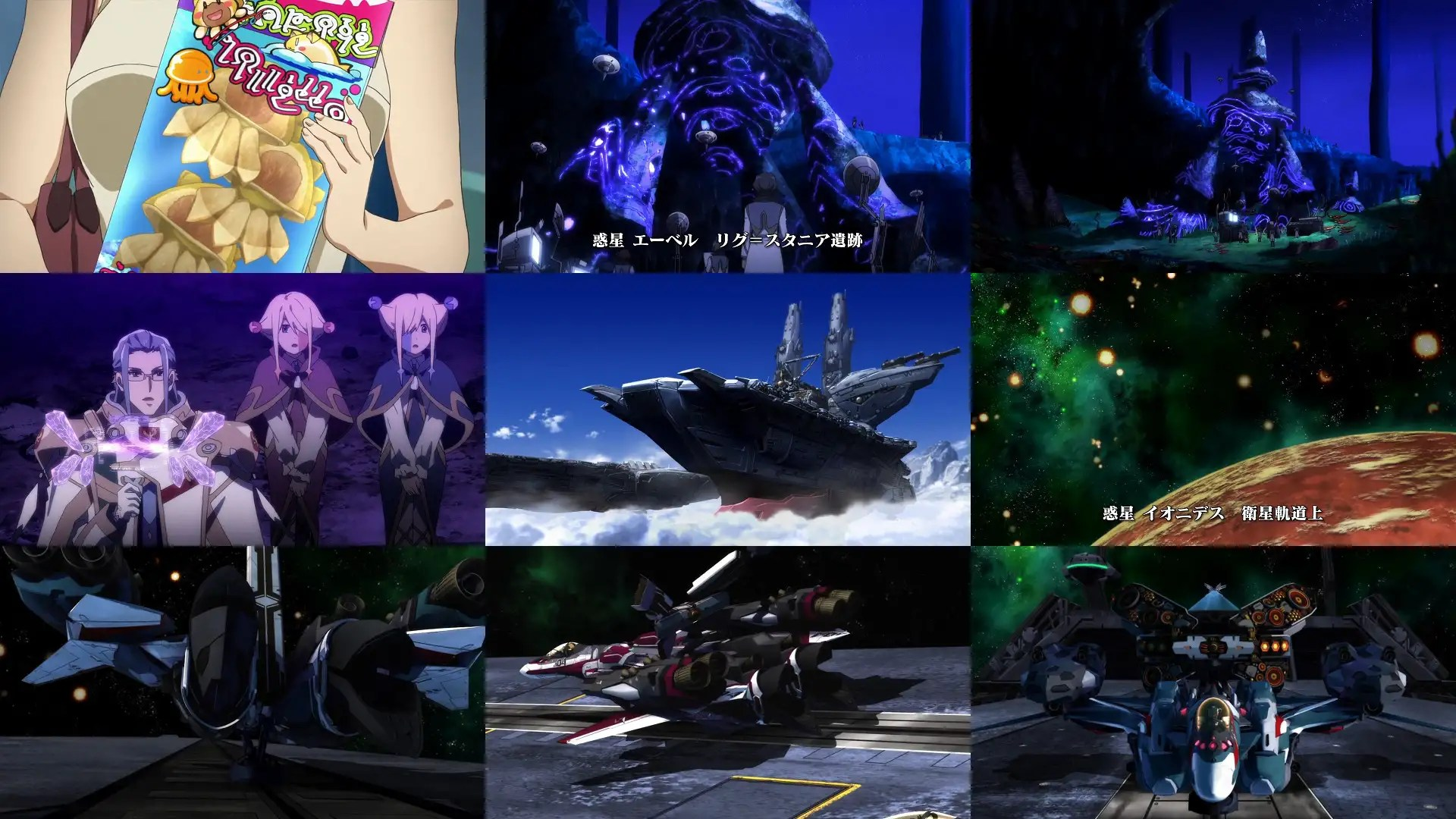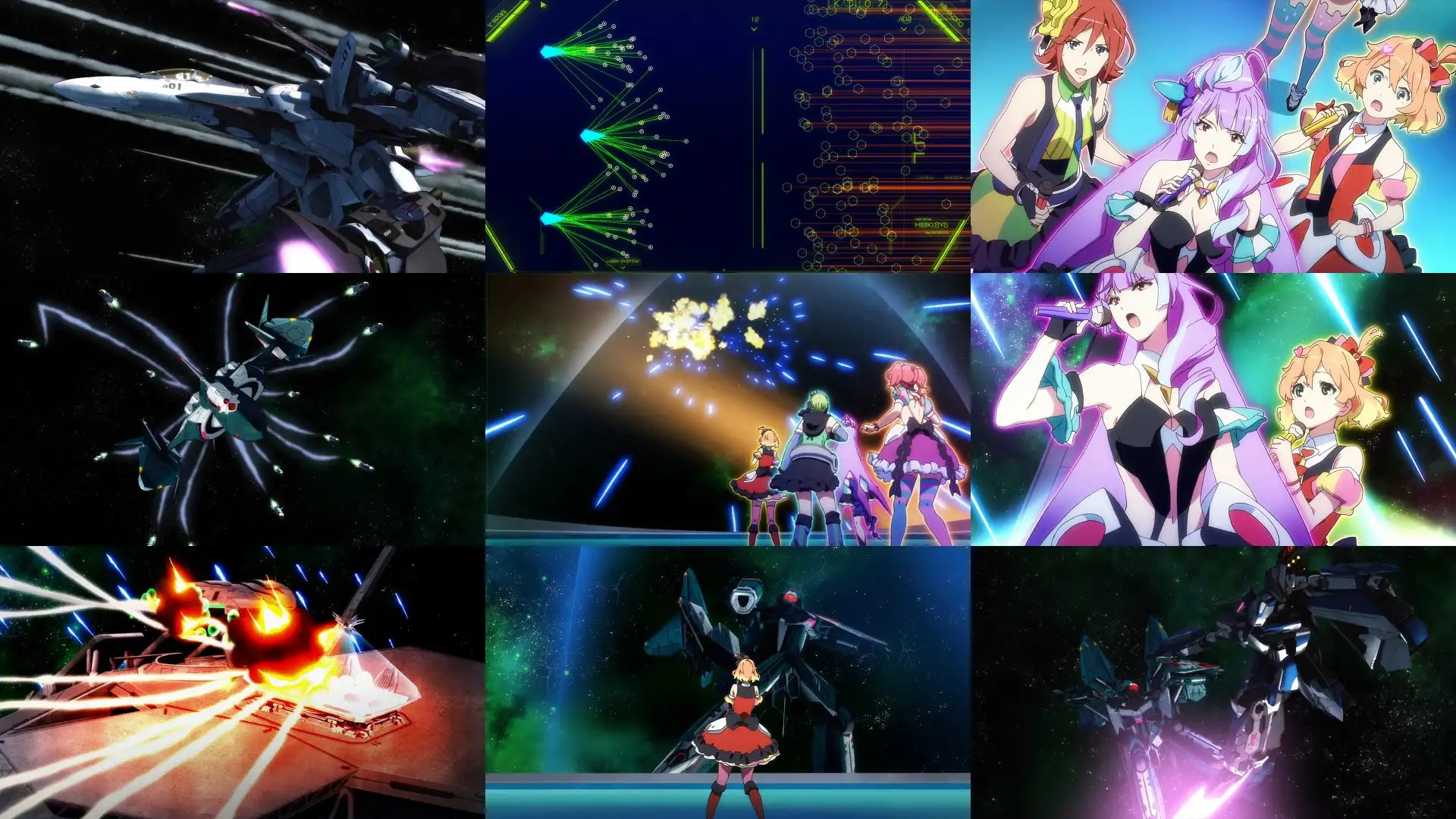4+3+3+4+4 3+3+3+3+3=33「Macross Delta 007」惑星ヴォルドール回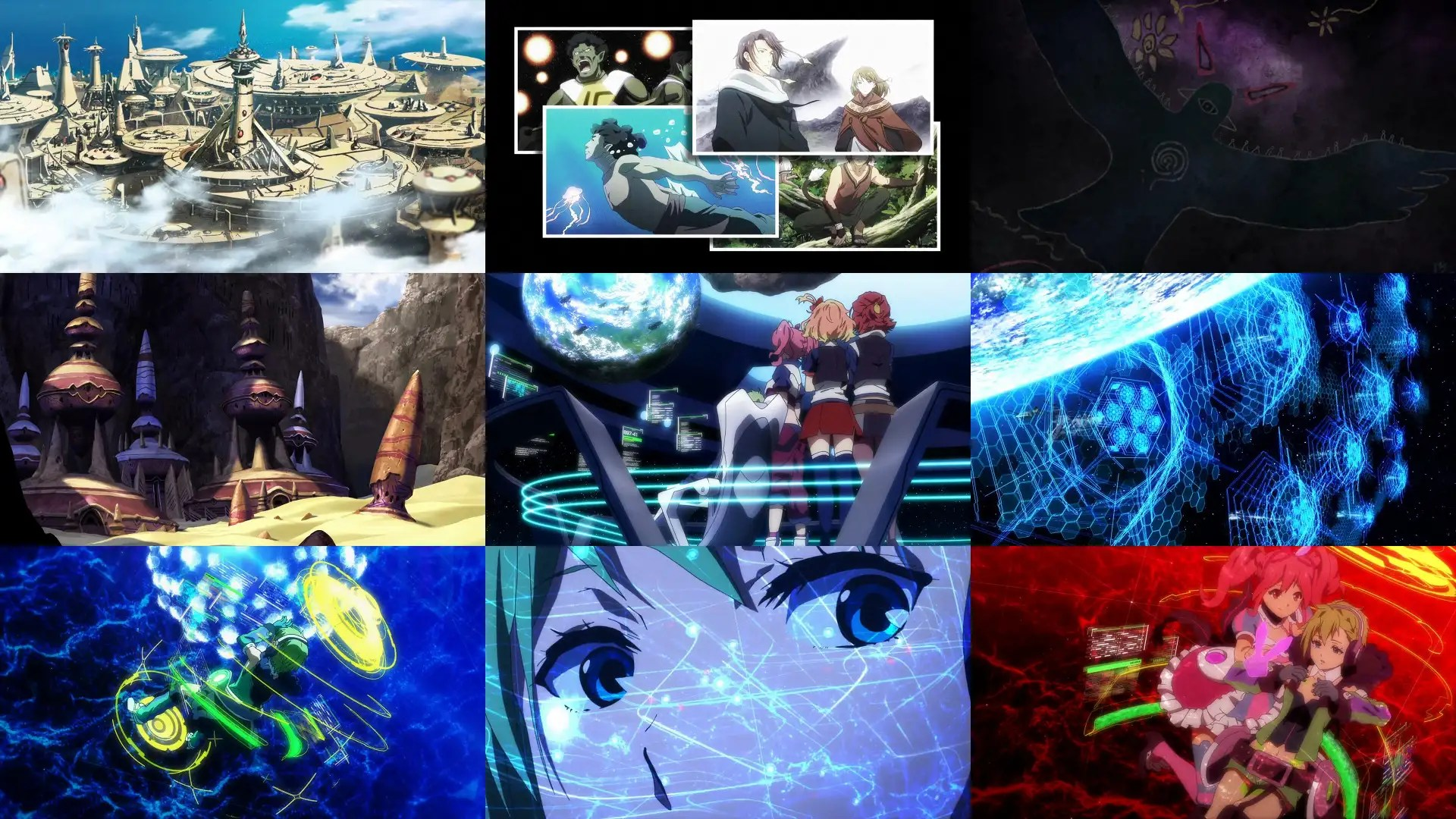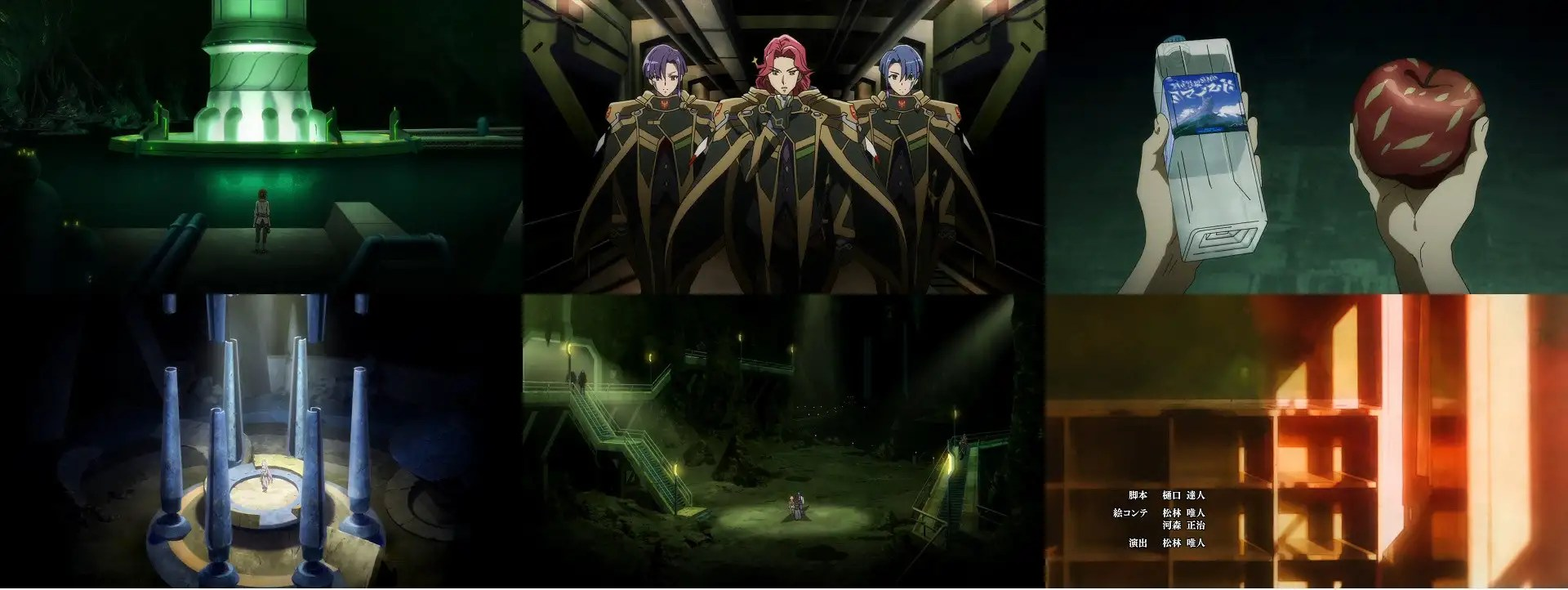3+4+3+5+3 4+4+3+3+3=35「Macross Delta 008」確定「為何而戰？」回

Psycho-Pass第二季及2015最新的攻殻機動隊 ARISE中，也都有全息影像混亂判斷的橋段。但過程描寫，令人覺得非常有道理，這就是因腳本條理營造的真實感差別。

ワルキューレ小隊除了歌唱精神攻擊外，幻象也有混亂敵人心智的效果www

ランカ・リー的「アイモ～鳥のひと」戰場版，因應殺生內心矛盾劇情，影音帶給觀眾相當強烈的不快感。是完美的詮釋。相較フレイア的聲優其實相當不錯，從表現可看出她的努力，聲質近似中島愛，歌唱力更上一層。但角色缺乏經典場面及內心戲表現，是比ランカ・リー吃虧之處。4+4+4+4+3  3+3+3+3+3=34「Macross Delta 009」メッサー個人回。
マクロス系列作中，時常會出現一個犧牲的男性角色，這代八成就是這位メッサー了www   兩位女角站在ハヤテ兩旁各懷思慮的鏡頭不錯，敵方子機分離戰鬥的部分也不錯。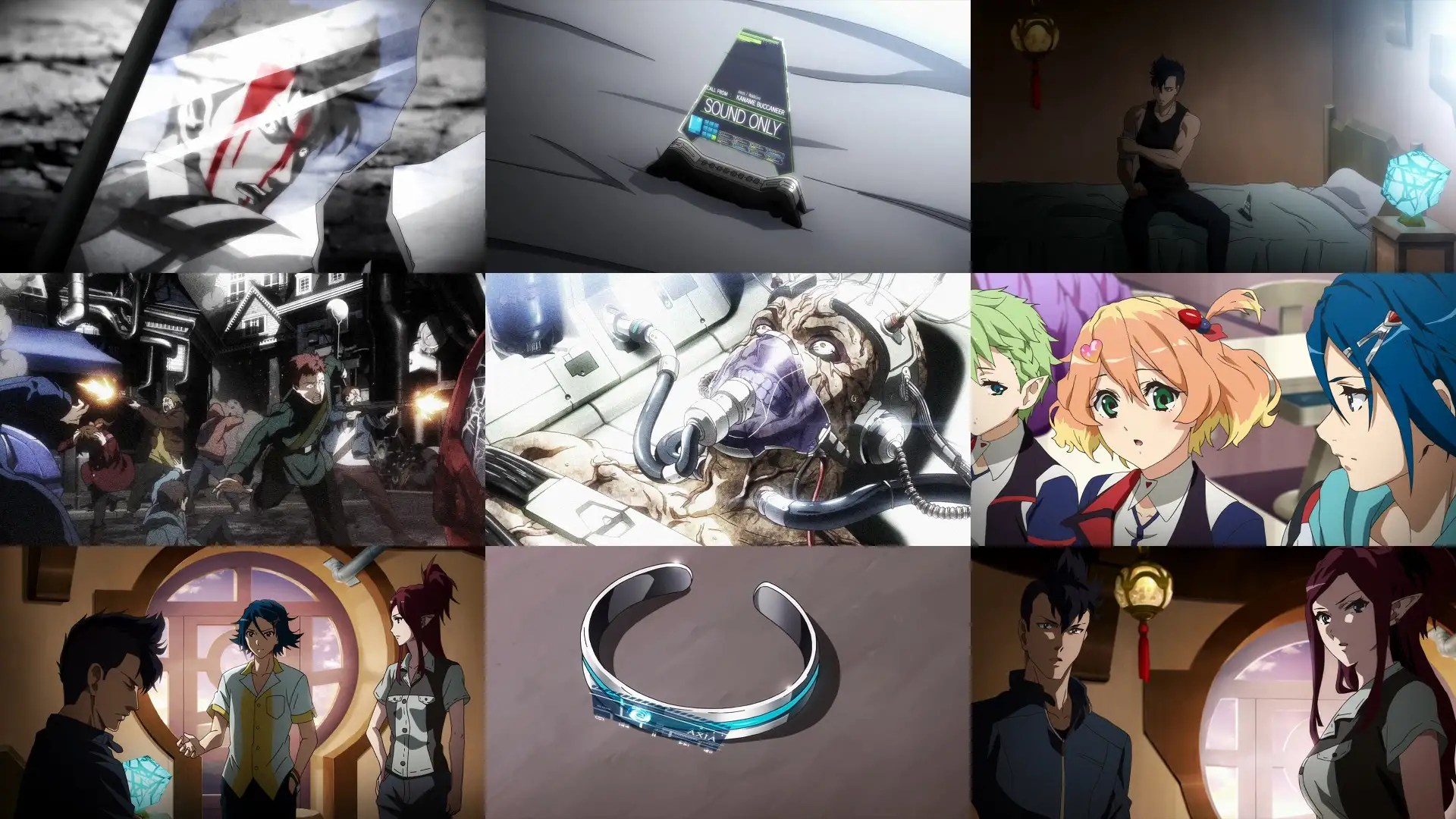4+4+3+3+3  3+5+3+3+3=32「迷家‐マヨヒガ‐ 009」多頭行動，光宗確定心意回。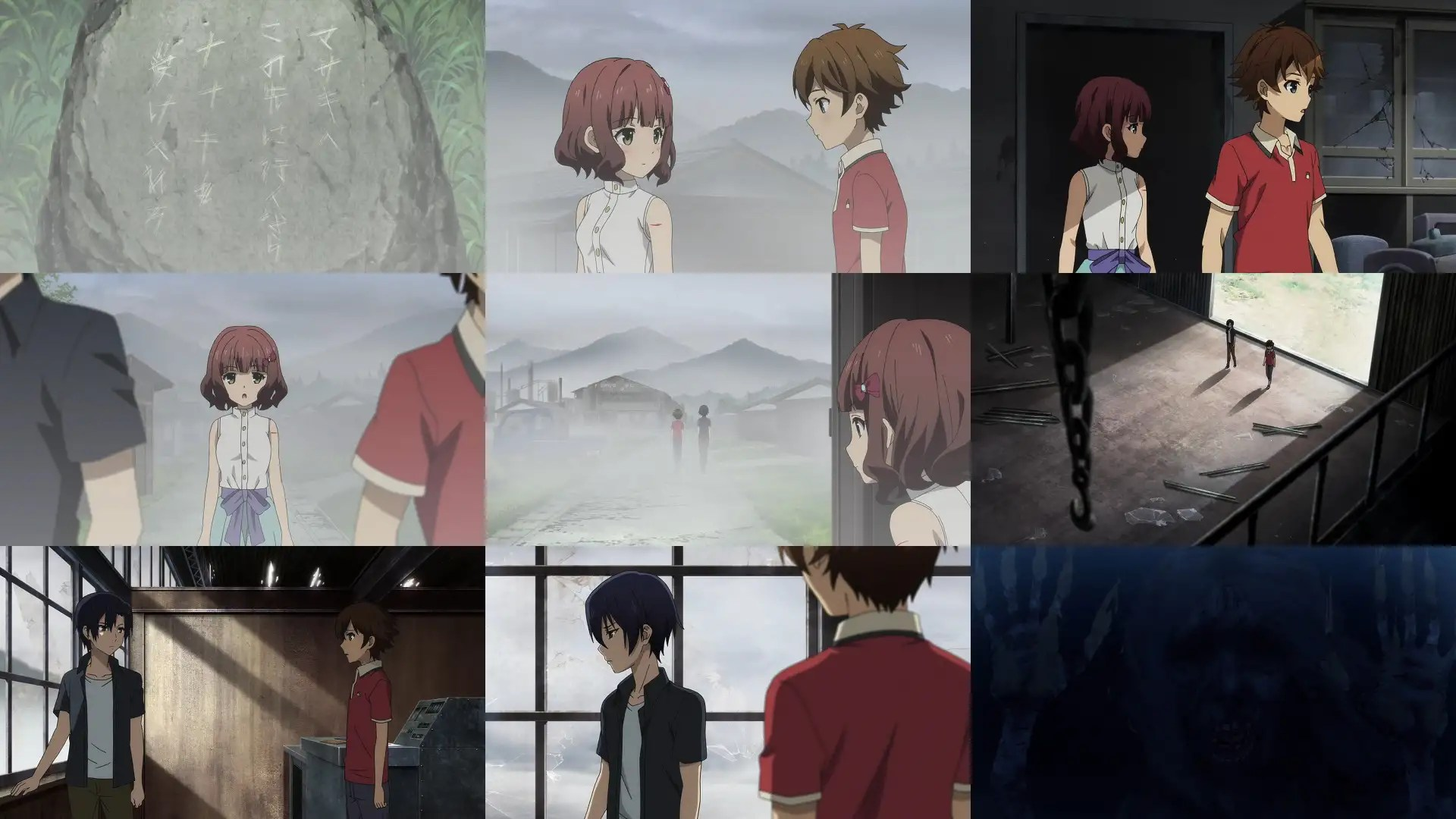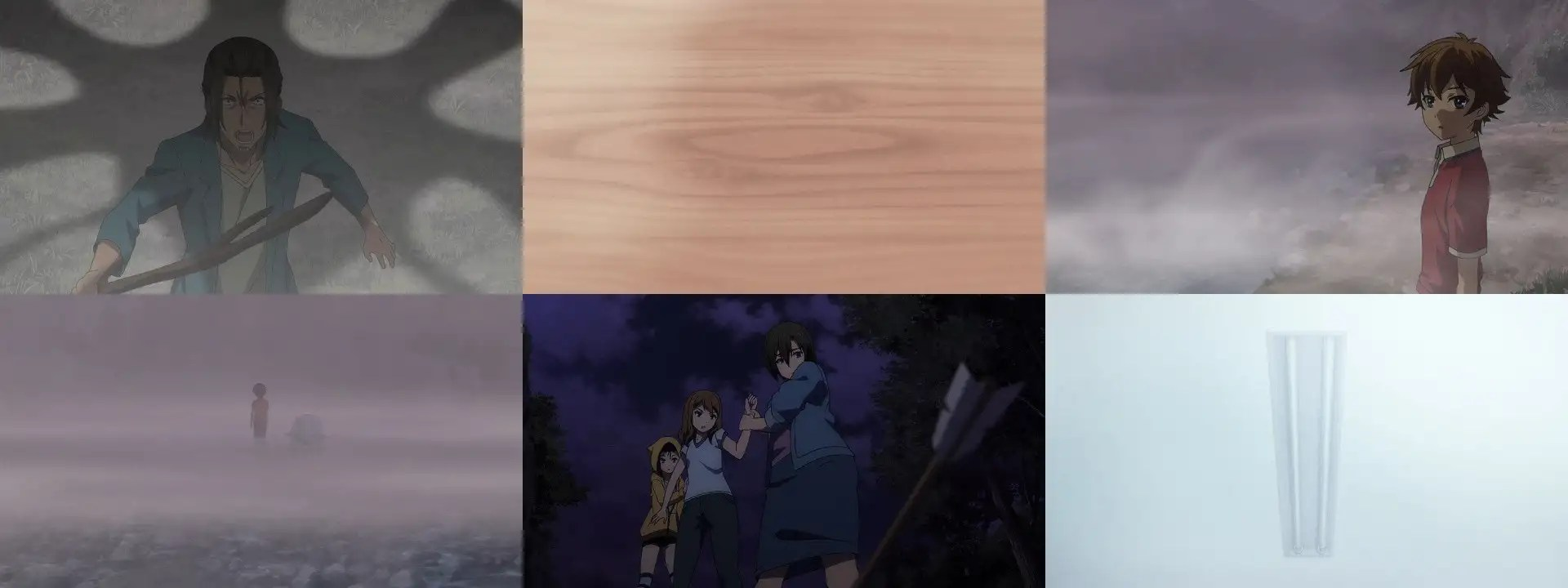4+3+3+4+4  3+4+3+3+3=34「リルリルフェアリル〜妖精のドア〜 017」

ROSE可惜事件後續沒有個人心得感想，哥倆於事件後也沒有新的一番認知。僅止於意氣相投與否之描述。

ROSE很有趣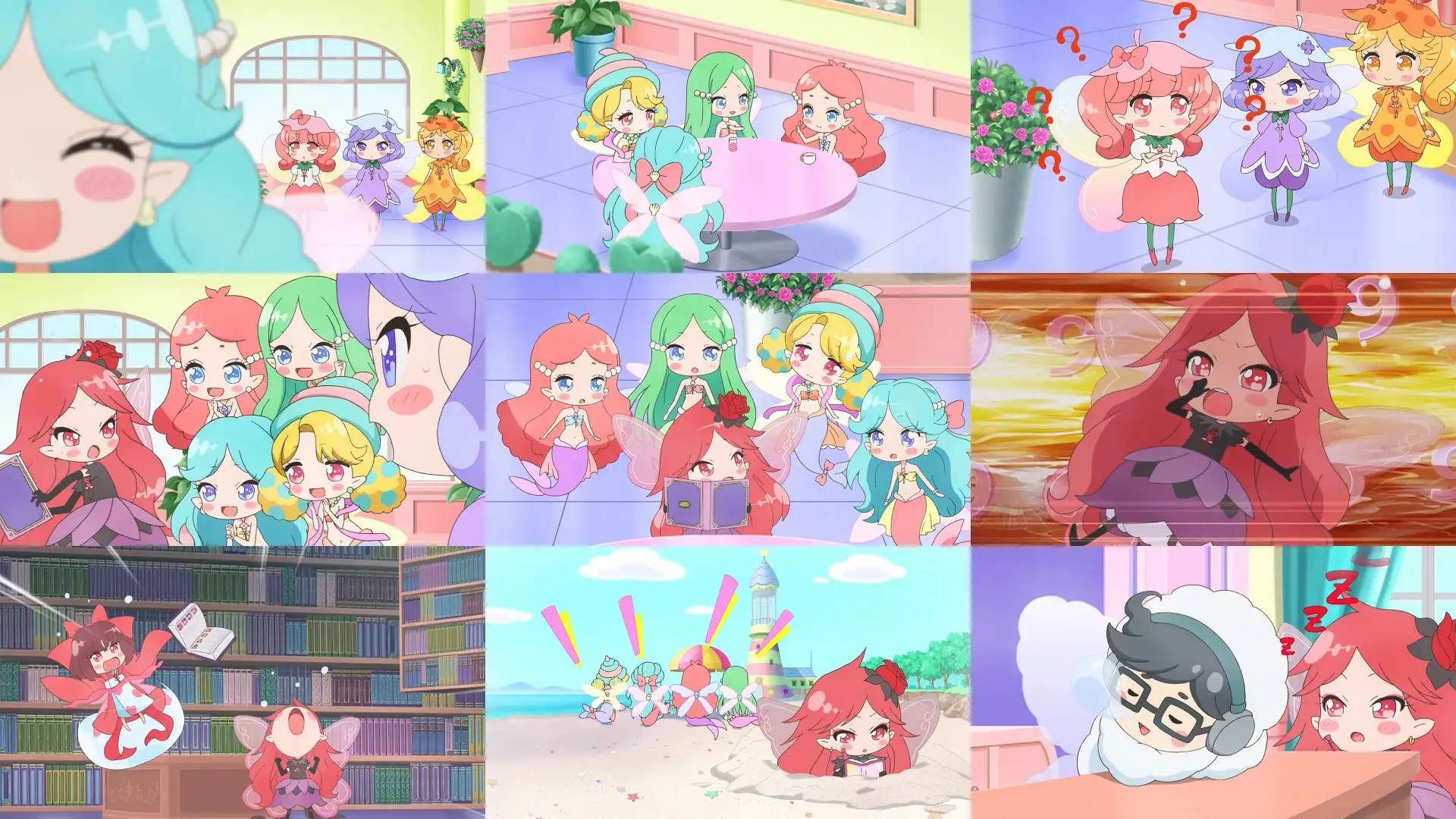ROSE算是反差萌類型角色4+4+3+3+4 3+3+3+3+3=33「 プリパラ S3 009 (098)」後生可畏回。

3DCG部分，在影像細節上，本季也稍微劣化了點。究竟是怎麼回事呢？不是聽說プリパラ的大型機台很賺嗎？

カノン的新曲倒是還不錯啦，熱鬧且不失可愛的曲子。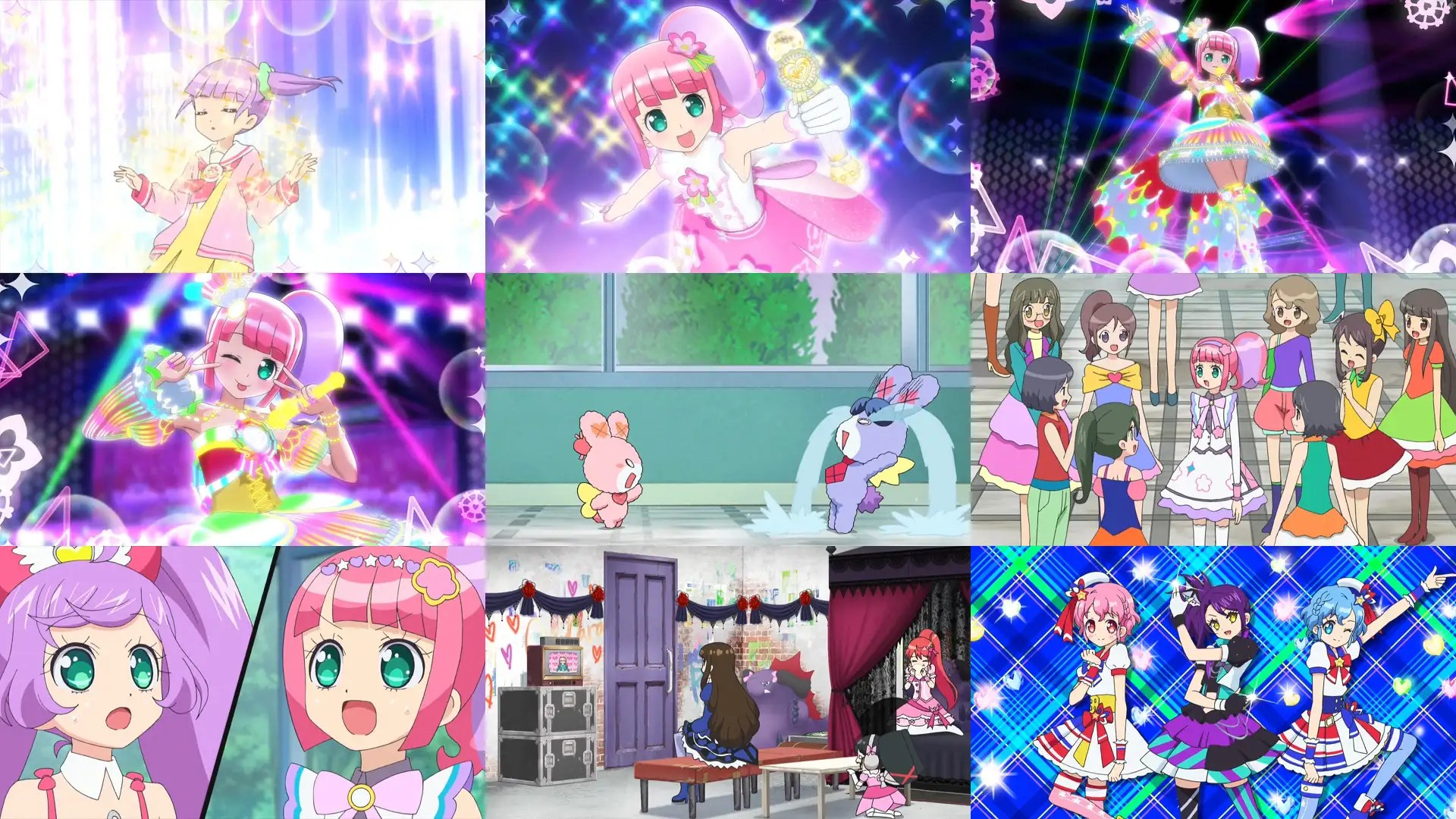らぁら妹妹，很有日語所謂的 (腹黑) 潛力。所幸出發點單純，心態尚且不到作惡的程度。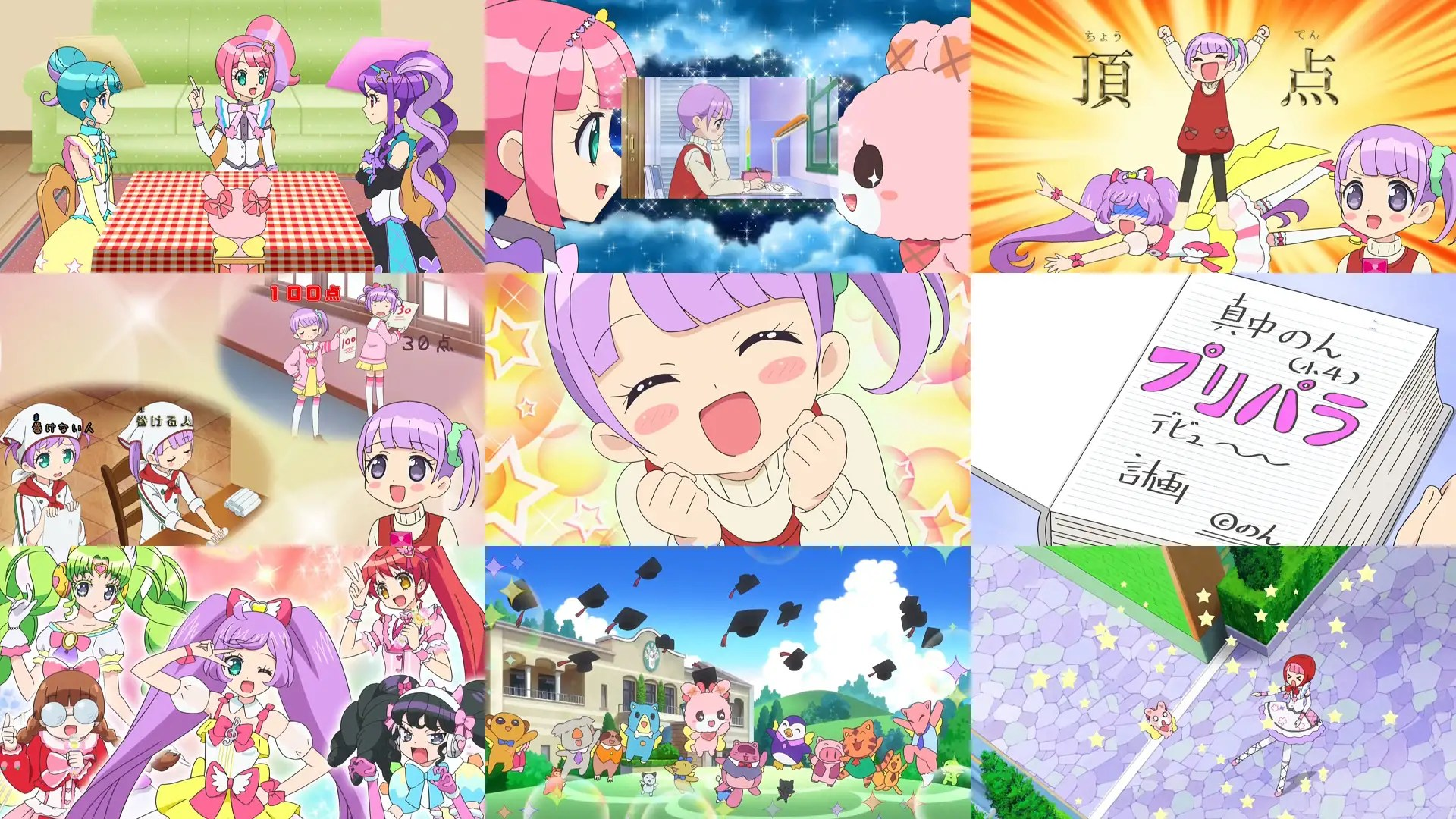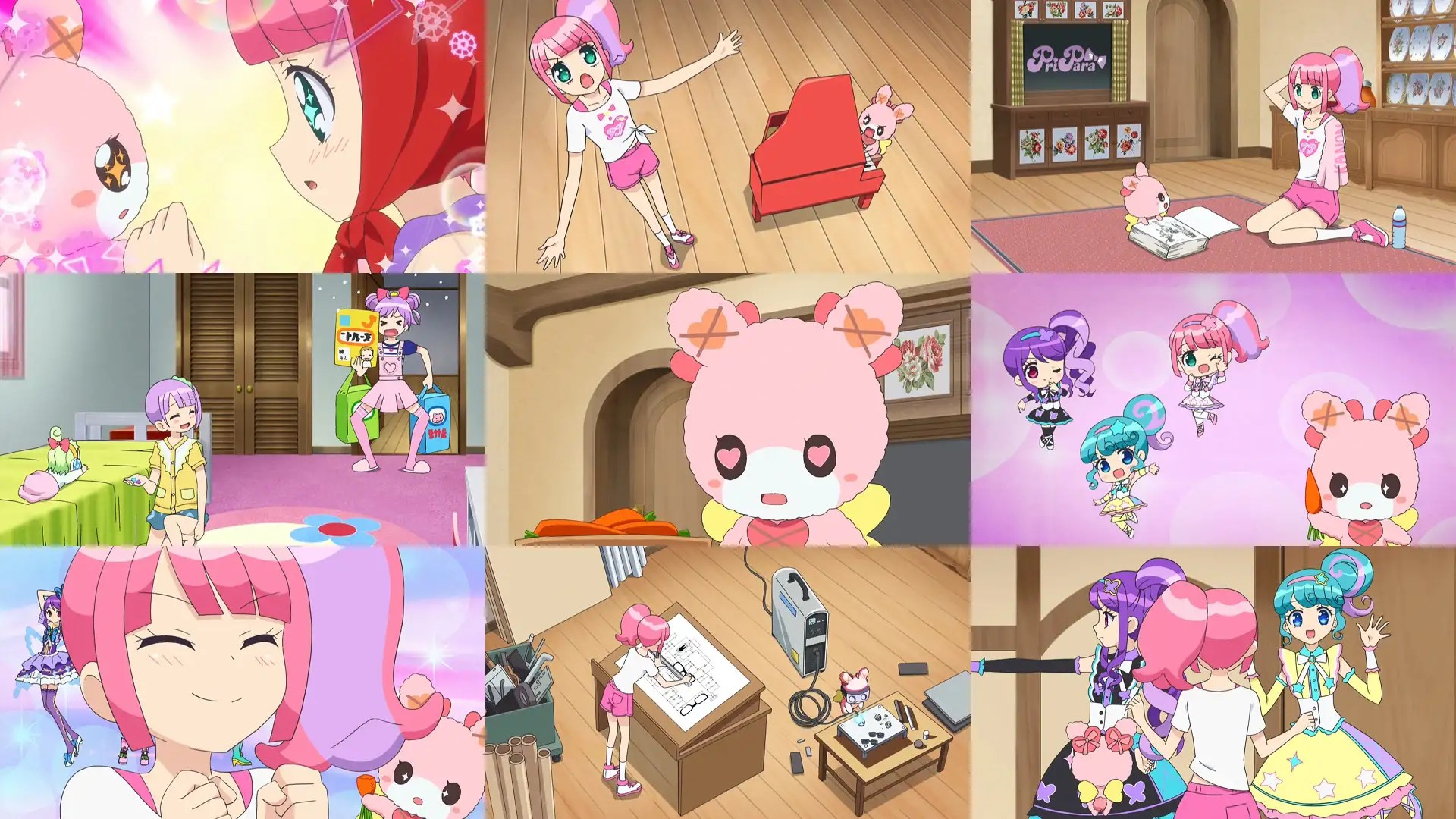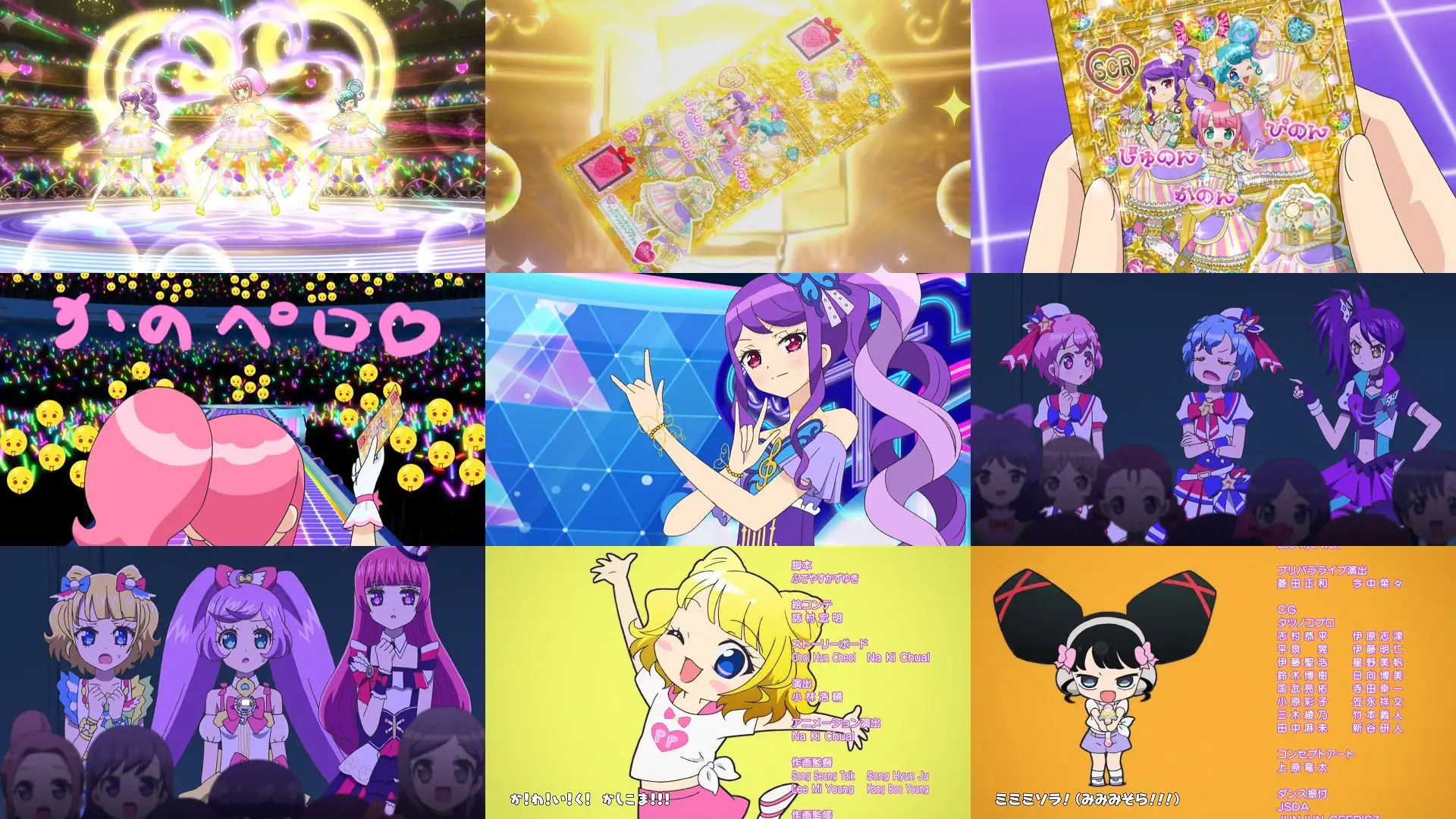4+3+3+3+3(+-1)  3+3+3+3+4=32「迷家‐マヨヒガ‐ 010」關於精神及肉體的一話。

E項+-1的理由，
+1 的理由是，父親回憶及教授回憶兩橋段啣接自然順暢。E項節奏：橋段啣接
– 1 的理由是，父親會面轉教授研究私室場景，換場換幕不自然。剛剛才哭著見面，忽然老爸就不見了。時間感模糊。

「 マサキ ナナキ レイジ」三字可各自寫成「魔(の)先 名無き 霊示」分別代表，負面引導，心魔，正向引導。算是額外的文字遊戲。
「真咲」這人也的確奇怪，仔細回頭看一遍第一集，會發現其實從第二集以後，性格改變很多。感情豐富多話，變感情平靜寡言。好像不同個人似的。マイマイ是不錯的角色。角色講出在它情境經驗下，自我思考的話語。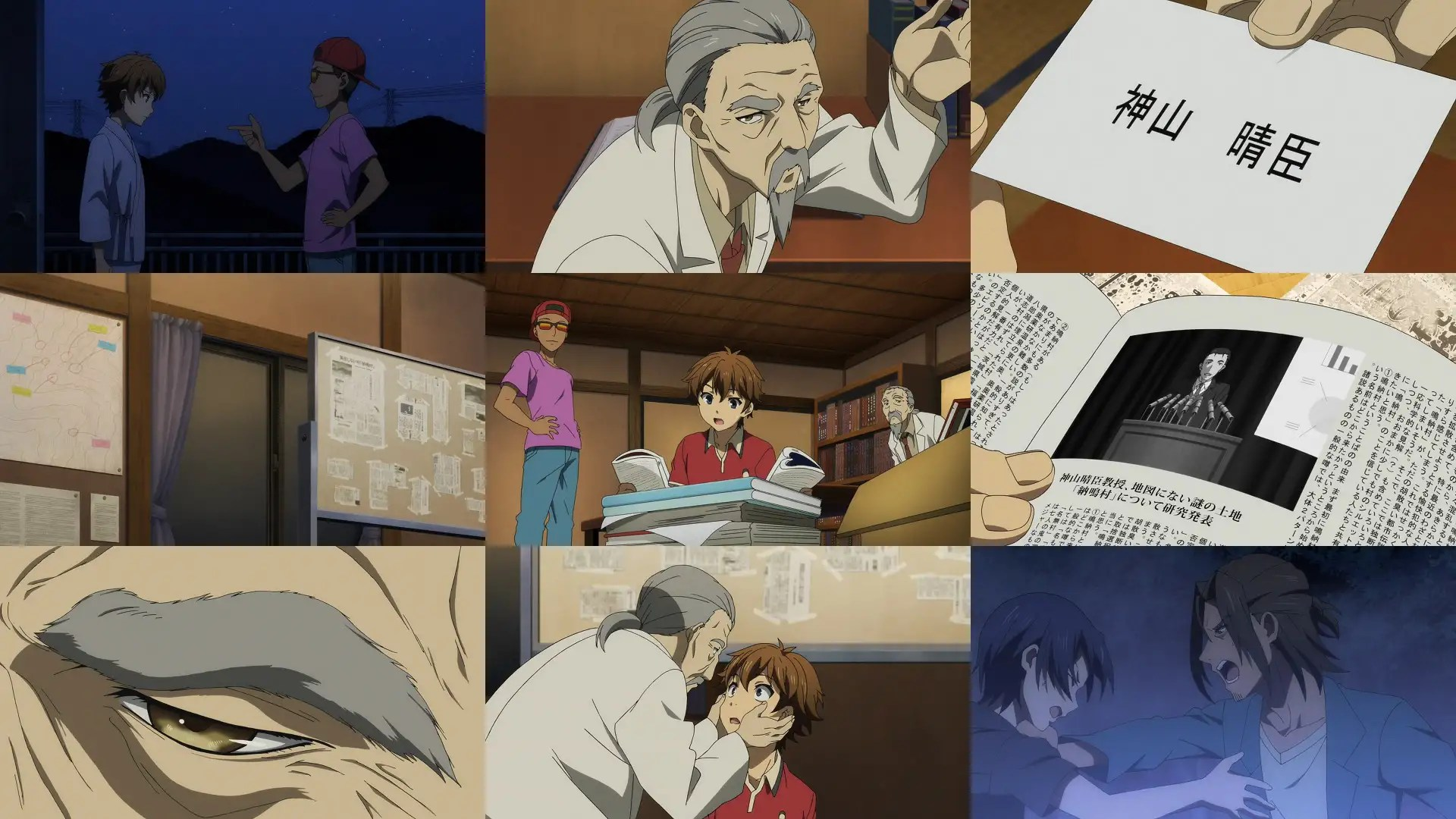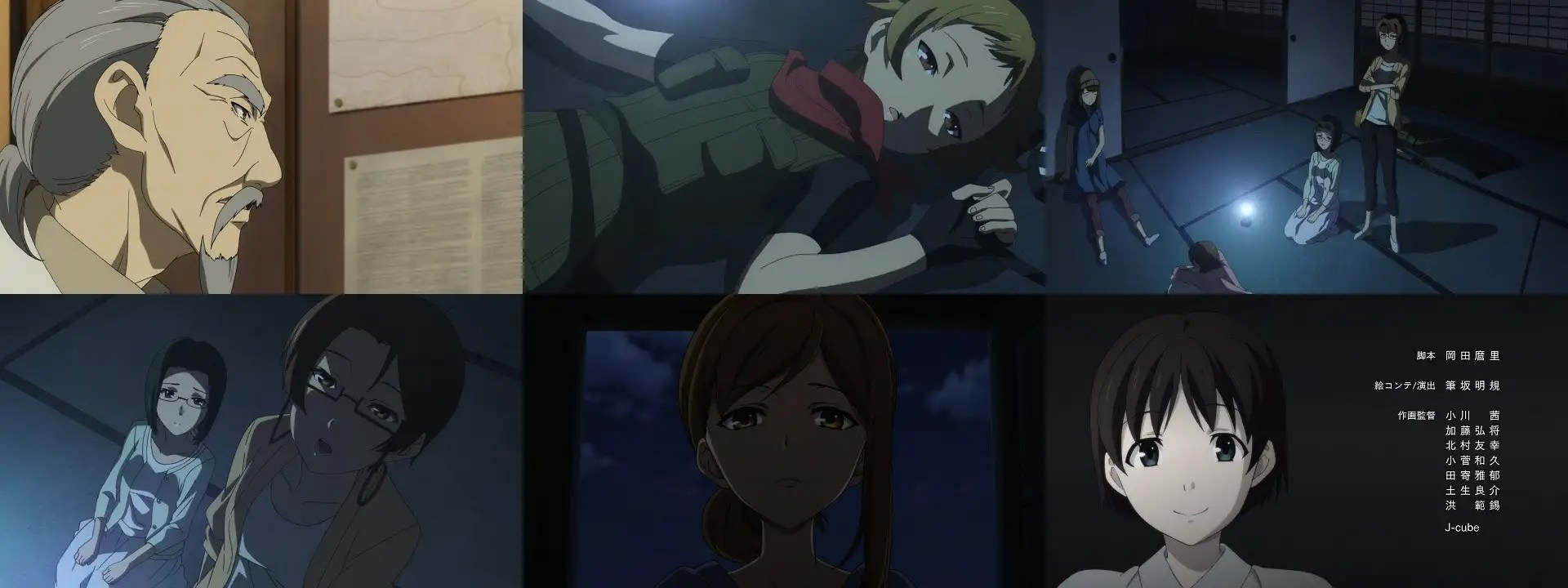## 發表迴響 »

This site uses Akismet to reduce spam. Learn how your comment data is processed.